#### You may also like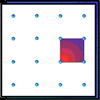### Geoboards

This practical challenge invites you to investigate the different squares you can make on a square geoboard or pegboard.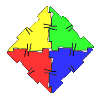### Polydron

This activity investigates how you might make squares and pentominoes from Polydron.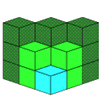If you had 36 cubes, what different cuboids could you make?

# Family Tree

##### Age 7 to 11Challenge Level

We had several solutions to this problem. Michael explained his reasoning as below. You will probably find it easiest to follow the solution if you fill in the people on a copy of the tree as you read it.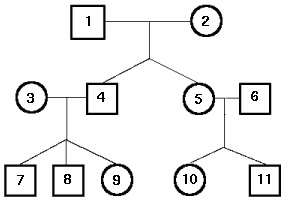G must be $1$ or $2$ as she mentions a granddaughter; and she is female so she is number $2$. We also know that she is a non-mathematician. Her granddaughter, B, must be $9$ or $10$.

E, as the youngest male, must be $8$ or $11$.

J is $6$ or $3$ (if we allow 'brother-in-law' to describe her husband's sister's husband).

F has exactly one brother, so must be $5$, $7$, $8$ or $10$.

C has a husband, and says her father is a mathematician, so C is $5$. Therefore $1$ is a mathematician, and as the oldest mathematician in the family can be identified as I. F can no longer be $5$.

D, having cousins in the tree, must be one of the third generation.

There is now no option but to assume that J is $6$. [Michael hasn't quite explained why: he probably noticed that $6$ is C's husband, and therefore not a mathematician, and so J can't be $3$.] From here we can deduce that $4$ is a mathematician, and that E and B are his children, since they have each said that their father is a mathematician.

We can now rule out F being $7$ or $8$, and so F is $10$. F tells us that her parents (C and J) are not mathematicians, and her brother ($11$) is.

H is a mother of two mathematicians, so she is $3$, and either $7$ or $9$ is a mathematician (we were told that E is). This means that for D to have just one mathematician cousin, D must be $7$ and F must be a non-mathematician.

Finally, A must be $11$, as his father is a mathematician, so K is $11$. We still haven't said which of $7$ and $9$ is the mathematician, but since we haven't yet got a female mathematician, it must be B.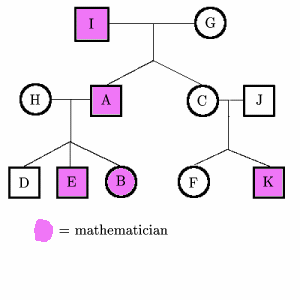Other people who sent in correct solutions (but mostly without such detailed explanation) were Claire , Sian and Fiona of Stamford High School; Ankaru; Samantha, Jacqui, Sandy and Claire of The Mount School, York; Chong Ching, Clement Goh, Chen Wei and Ng Yan; Emma and Monica of Hethersett High School, Norwich.